# 2. Determine the vertical displacement at joint B and horizontal displacement at joint D using Castigliano's...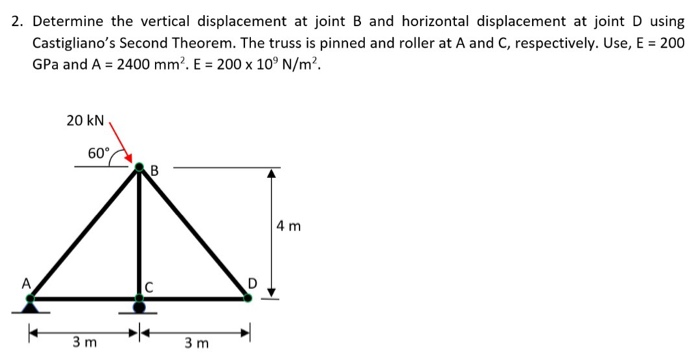2. Determine the vertical displacement at joint B and horizontal displacement at joint D using Castigliano's Second Theorem. The truss is pinned and roller at A and C, respectively. Use, E = 200 GPa and A = 2400 mm. E = 200 x 10°N/m². 20 KN 60 CAB 4 m 3m 3m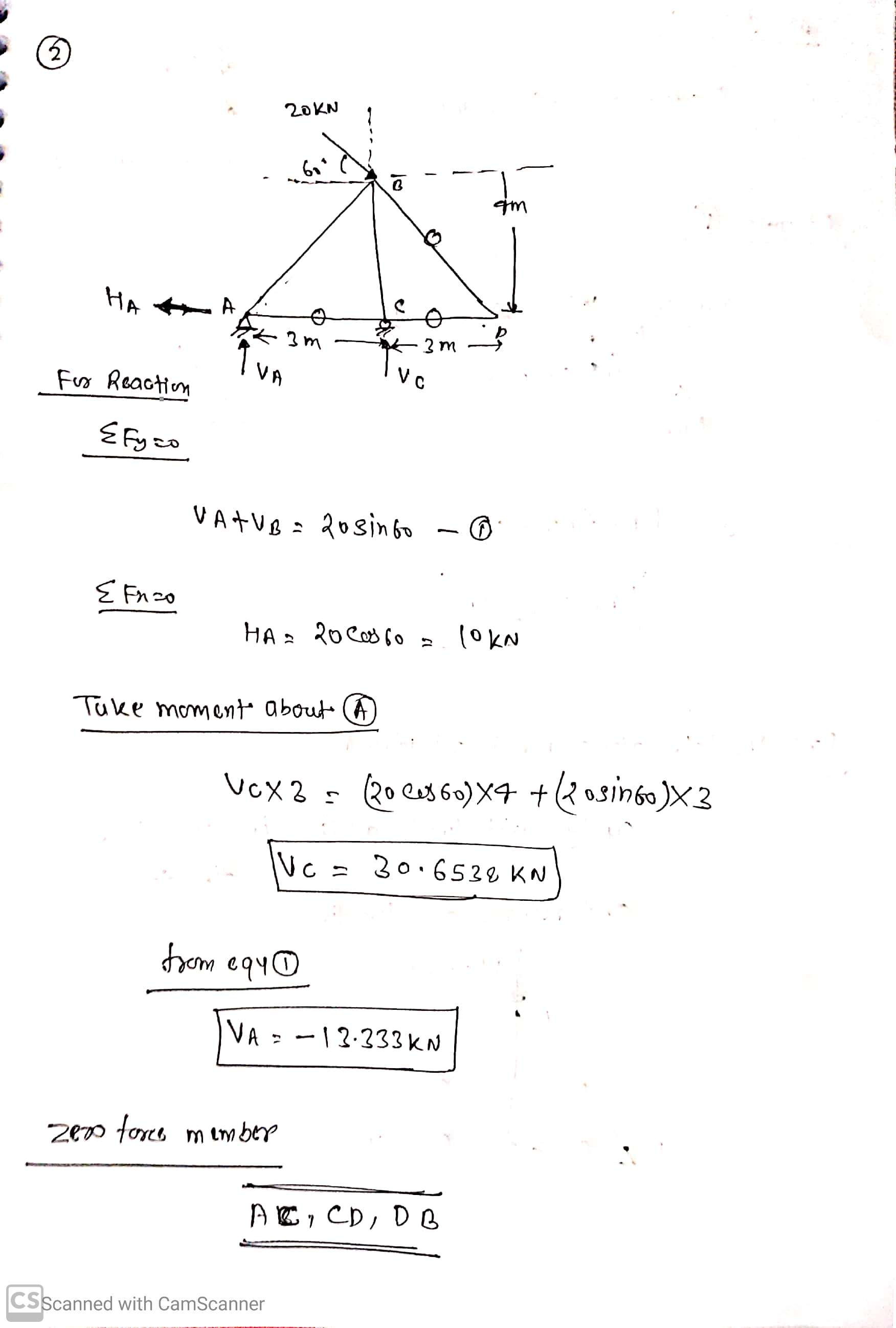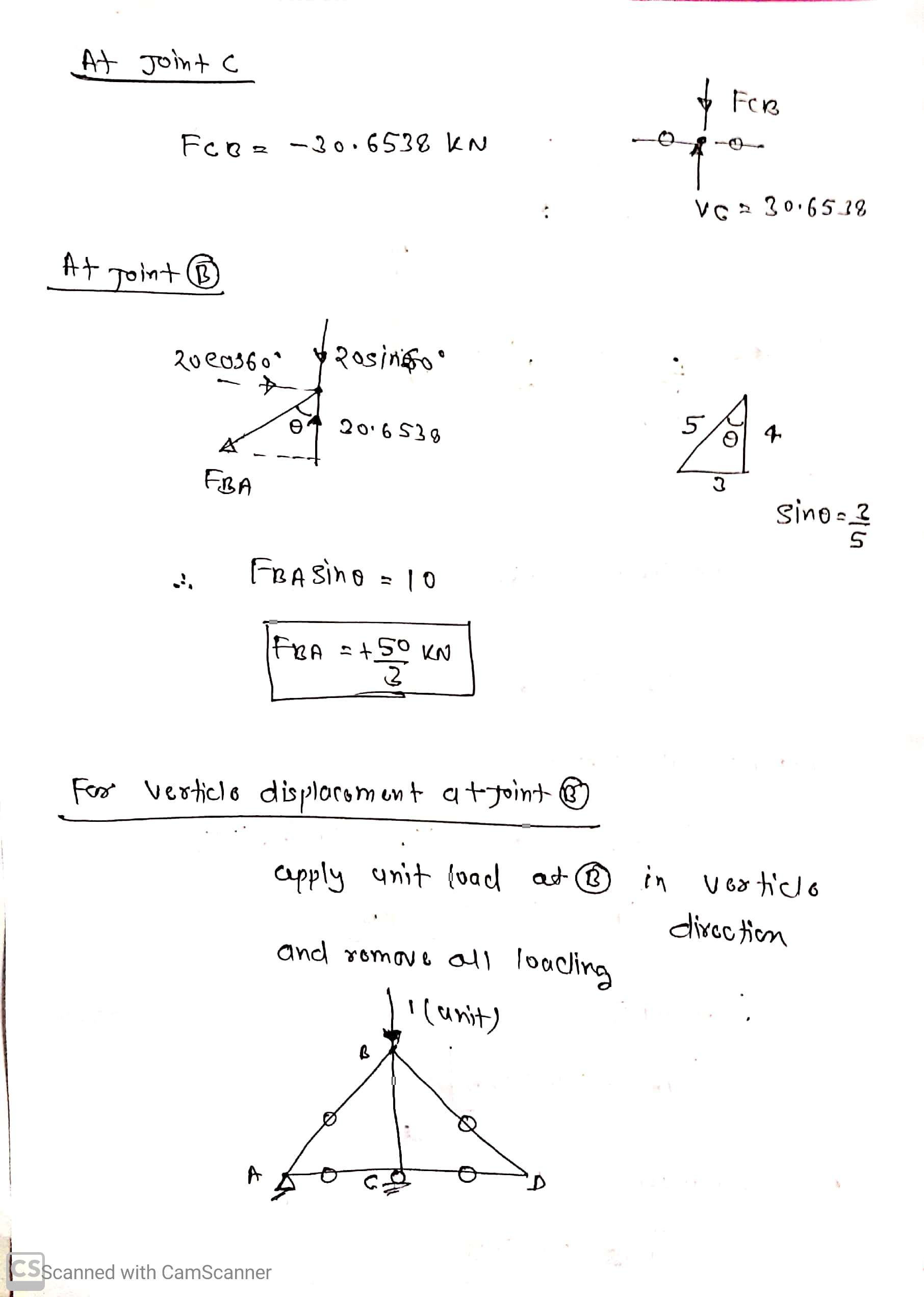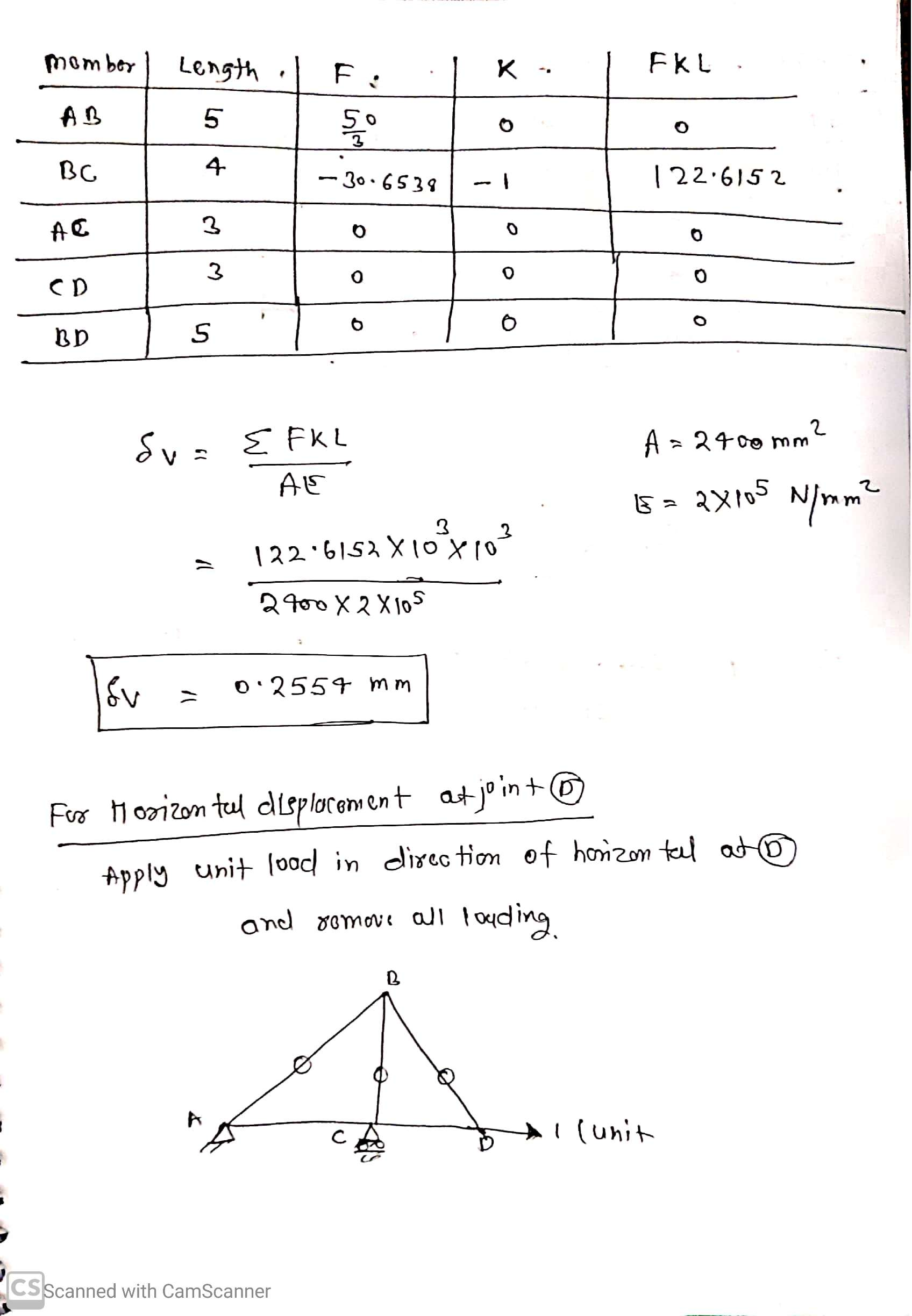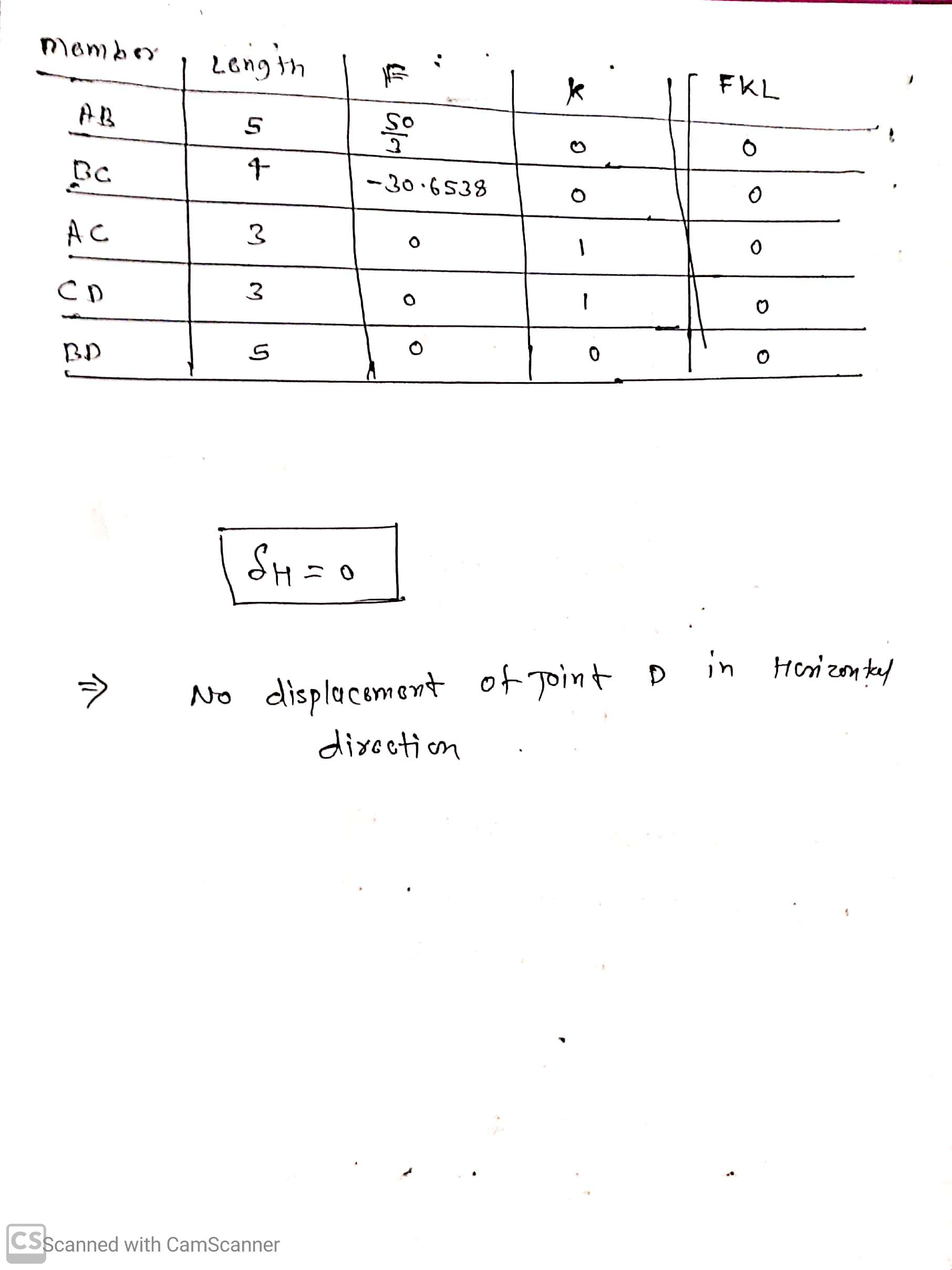#### Earn Coin

Coins can be redeemed for fabulous gifts.

Similar Homework Help Questions
• ### Please show all work 4 kN 2. Use Castigliano's theorem to find the vertical displacement of...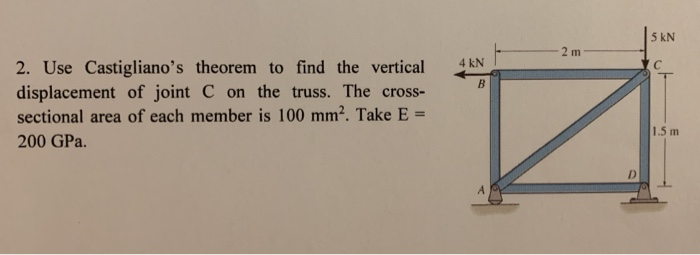Please show all work 4 kN 2. Use Castigliano's theorem to find the vertical displacement of joint C on the truss. The cross- sectional area of each member is 100 mm2. Take E = 200 GPa. 1.5 m

• ### Q3: Determine the vertical and horizontal displacement of joint A for the truss shown in Fig....Q3: Determine the vertical and horizontal displacement of joint A for the truss shown in Fig. (3). each bar is made of steel and has the cross-sectional area of 400mm Take E = 200 GPa Use the method of virtual work. E D 2 m |в -1.5 m -1.5 m 20 KN 40 KN Fig. (3)

• ### Q3: Determine the vertical and horizontal displacement of joint A for the truss shown in Fig....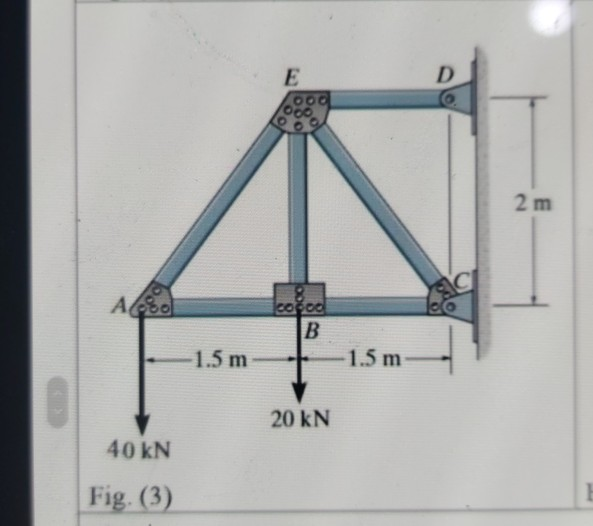Q3: Determine the vertical and horizontal displacement of joint A for the truss shown in Fig. (3), each bar is made of steel and has the cross-sectional area of 400mm?. Take E = 200 GPa. Use the method of virtual work. E D 00. 2 m СІ Ao cocoa B 1.5 m 1.5 m 20 KN 40 kN Fig. (3)

• ### 4. Determine the vertical displacement of point C using Castigliano's theorem. Include axial displacements. Assume E,...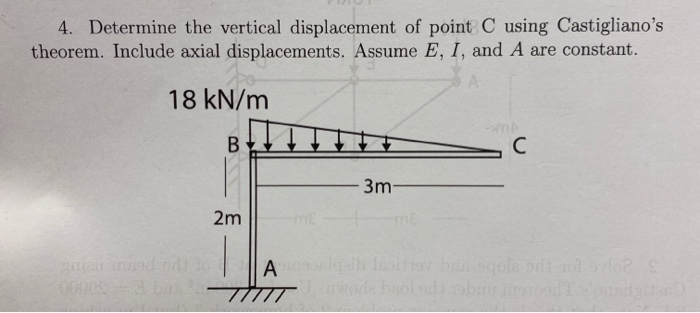4. Determine the vertical displacement of point C using Castigliano's theorem. Include axial displacements. Assume E, I, and A are constant. 18 kN/m 3m- 2m Alle

• ### Solve It fast Please Q3: Determine the vertical and horizontal displacement of joint A for the...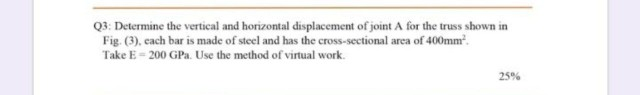Solve It fast Please Q3: Determine the vertical and horizontal displacement of joint A for the truss shown in Fig. (3). cach bar is made of steel and has the cross-sectional area of 400mmº. Take E = 200 GP. Use the method of virtual work. 25% E OO D 2 m AAS ered B -1.5 m -1.5 m 20 KN 40 KN Fig. (3)

• ### 2. (20 points) Using the unit load method (virtual work), find the horizontal displacement of node...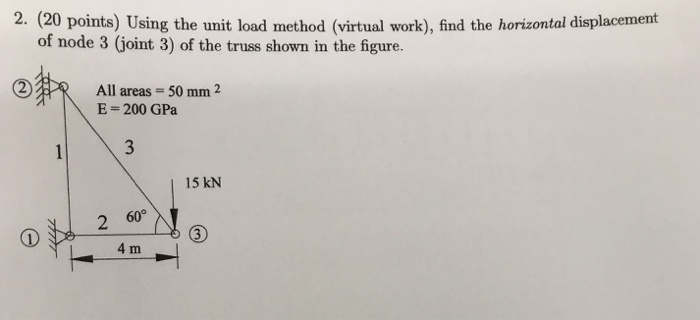2. (20 points) Using the unit load method (virtual work), find the horizontal displacement of node 3 (joint 3) of the truss shown in the figure. ? R All areas = 50 mm 2 E = 200 GPa 15 kN 2 60 L4 m

• ### i need the answer quickly fast as you can Q3: Determine the vertical and horizontal displacement...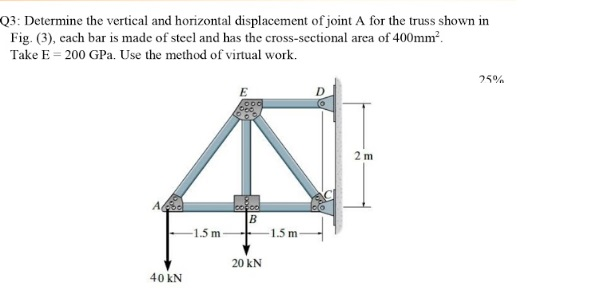i need the answer quickly fast as you can Q3: Determine the vertical and horizontal displacement of joint A for the truss shown in Fig. (3), cach bar is made of steel and has the cross-sectional area of 400mm? Take E = 200 GPa. Use the method of virtual work. 25% E 2 m А. cool B 1.5 m -1.5 m 20 KN 40 KN

• ### Question 1 (25 points) Determine the horizontal displacement of point C. Assume the members are pin...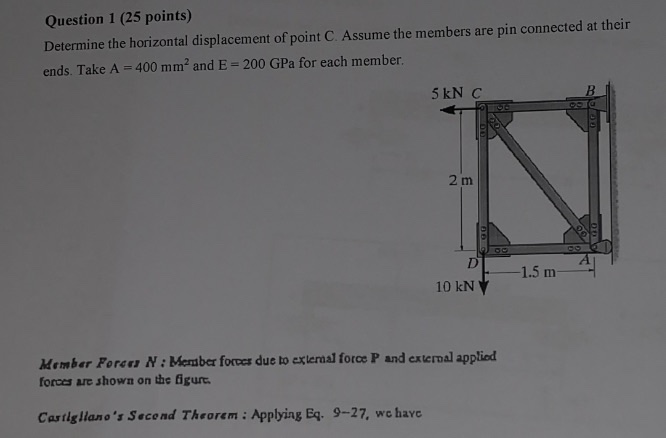Question 1 (25 points) Determine the horizontal displacement of point C. Assume the members are pin connected at their ends. Take A = 400 mm? and E = 200 GPa for each member. 5 kN C 2 m 1.5 m 10 kN Member Foron N: Member forces due to external force P and external applied forces are shown on the figurt. Castigliano's Second Theorem : Applying Eq. 9-27, we have

• ### SOLVE B AND C USING CASTIGLIANO'S THEOREM Consider the cantilever beam shown in . Suppose that...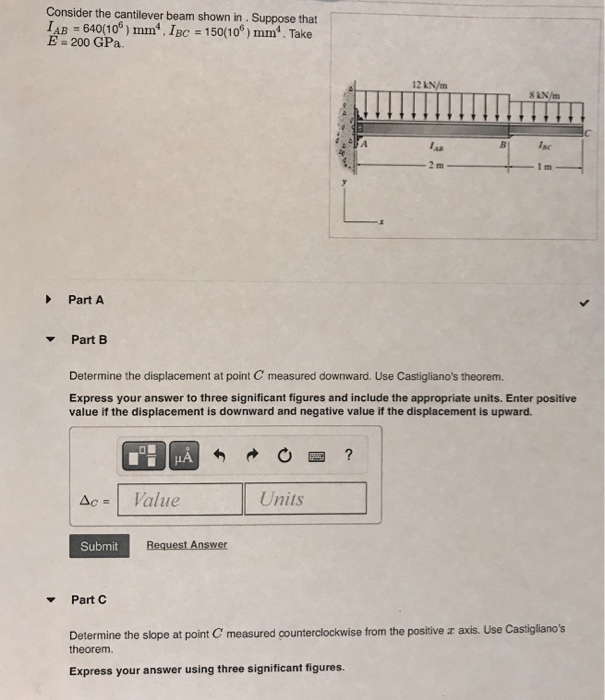SOLVE B AND C USING CASTIGLIANO'S THEOREM Consider the cantilever beam shown in . Suppose that LAB = 640(106) mm IBC = 150(106) mm". Take E 200 GPa 12 kN/m 8 kN/m موا Inc Part A Part B Determine the displacement at point C measured downward. Use Castigliano's theorem. Express your answer to three significant figures and include the appropriate units. Enter positive value if the displacement is downward and negative value if the displacement is upward. HA ? Ac...

• ### Use the stiffness method to determine the horizontal and vertical components of the displacement at Joints...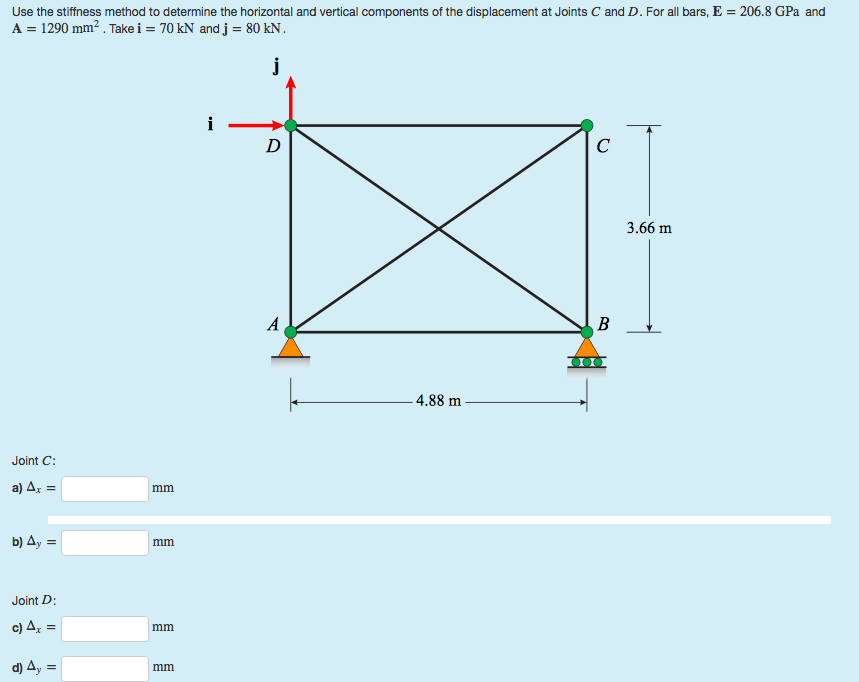Use the stiffness method to determine the horizontal and vertical components of the displacement at Joints C and D. For all bars, E = 206.8 GPa and A = 1290 mm². Take i = 70 kN and j = 80 kN. 3.66 m 4.88 m Joint C: a) Az = b) 4y = Joint D: c) 4, = d) 4, =